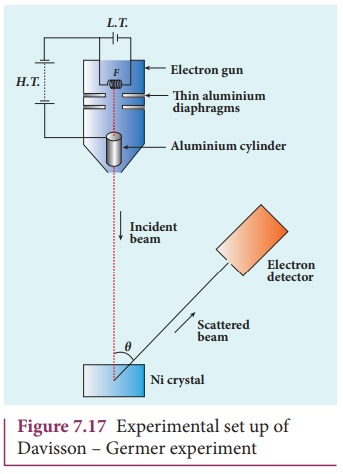Home | | Physics 12th Std | Davisson - Germer experiment

# Davisson - Germer experiment

De Broglie hypothesis of matter waves was experimentally confirmed by Clinton Davisson and Lester Germer in 1927.

Davisson - Germer experiment

De Broglie hypothesis of matter waves was experimentally confirmed by Clinton Davisson and Lester Germer in 1927. They demonstrated that electron beams are diffracted when they fall on crystalline solids. Since crystal can act as a three-dimensional diffraction grating for matter waves, the electron waves incident on crystals are diffracted off in certain specific directions. Figure 7.17 shows a schematic representation of the apparatus for the experiment.The filament F is heated by a low tension (L.T.) battery. Electrons are emitted from the hot filament by thermionic emission. They are then accelerated due to the potential difference between the filament and the anode aluminium cylinder by a high tension (H.T.) battery. Electron beam is collimated by using two thin aluminium diaphragms and is allowed to strike a single crystal of Nickel.

The electrons scattered by Ni atoms in different directions are received by the electron detector which measures the intensity of scattered electron beam. The detector is rotatable in the plane of the paper so that the angle Ď• between the incident beam and the scattered beam can be changed at our will. The intensity of the scattered electron beam is measured as a function of the angle Î¸.It is to be noted that electrons are not the only particles with which wave nature  can be demonstrated. The particles like neutrons and alpha particle are also associated with waves. They undergo diffraction when they are scattered by suitable crystals. Neutron diffraction studies are highly useful for investigating crystal structures.

Diffraction is one of the properties of waves. Whenever waves are incident on an obstacle, they bend around the edges of the obstacle. This bending of waves is called diffraction. The amount of bending depends on the wavelength of the waves.

We have learnt in unit 6 that as the wavelength of light is very small, diffraction effects of light are very small. In order to study diffraction of light, diffraction gratings are used.

Since X-rays and de Broglie waves of electrons have wavelengths (in the order of 10â€“10m) much shorter than that of the light wave, diffraction grating cannot be used for their diffraction. In a crystal, the spacing between atomic planes is comparable to the wavelength of x-rays and de Broglie waves of electrons. Hence, for their diffraction, the crystals are used which serve as three-dimensional grating.

Figure 7.18 shows the variation of intensity of the scattered electrons with the angle Î¸ for the accelerating voltage of 54V. For a given accelerating voltage V, the scattered wave shows a peak or maximum at an angle of 50Â° to the incident electron beam. This peak in intensity is attributed to the constructive interference of electrons diffracted from various atomic layers of the target material. From the known value of interplanar spacing of Nickel, the wavelength of the electron wave has been experimentally calculated as 1.65Ă….

The wavelength can also be calculated from de Broglie relation for V = 54 V from equation (7.18) asThis value agrees well with the experimentally observed wavelength of 1.65Ă…. Thus this experiment directly verifies de Broglieâ€™s hypothesis of the wave nature of moving particles.

Tags : Wave nature of particles | Physics , 12th Physics : UNIT 8 : Dual Nature of Radiation and Matter
Study Material, Lecturing Notes, Assignment, Reference, Wiki description explanation, brief detail
12th Physics : UNIT 8 : Dual Nature of Radiation and Matter : Davisson - Germer experiment | Wave nature of particles | Physics